Home   »   English Quizzes For SBI Clerk Mains...

# English Quizzes For SBI Clerk Mains 2022 – 19th December

Directions (1-15): In the following questions two columns are given containing three sentences/phrases each. In first column, sentences/phrases are A, B and C and in the second column the sentences/phrases are D, E and F. A sentence/phrase from the first column may or may not connect with another sentence/phrase from the second column to make a grammatically and contextually correct sentence. Each question has five options, four of which display the sequence(s) in which the sentences/phrases can be joined to form a grammatically and contextually correct sentence. If none of the options given forms a correct sentence after combination or none of them connects, mark (e), i.e. “None of the above” as your answer.

Q1.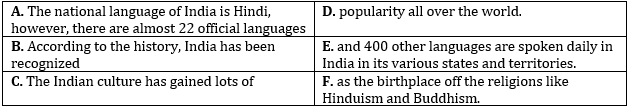(a) B-F
(b) A-E
(c) A-E and B-F
(d) C-D
(e) None of the above

Q2.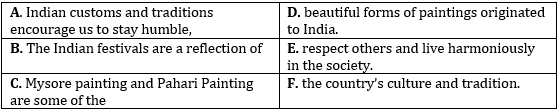(a) B-F
(b) A-E
(c) A-E and B-F
(d) C-D
(e) None of the above

Q3.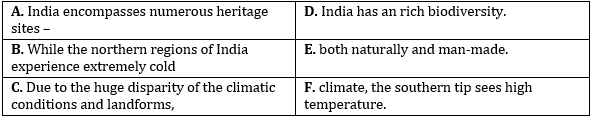(a) B-F
(b) A-E
(c) A-E and B-F
(d) C-D
(e) None of the above

Q4.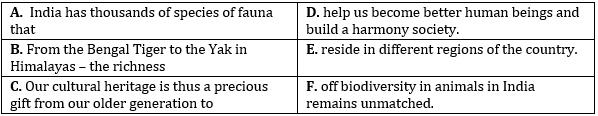(a) B-F
(b) A-E
(c) A-E and B-F
(d) C-D
(e) None of the above

Q5.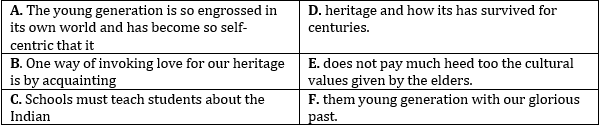(a) B-F
(b) A-E
(c) A-E and B-F
(d) C-D
(e) None of the above

Q6.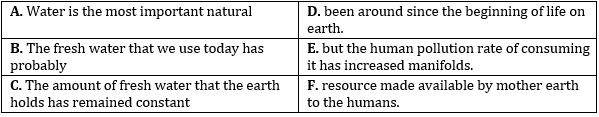(a) A-F, B-D and C-E
(b) A-F
(c) C-E
(d) C-E and B-D
(e) None of the above

Q7.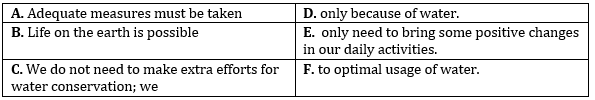(a) A-F, B-D and C-E
(b) A-F
(c) C-E
(d) C-E and B-D
(e) None of the above

Q8.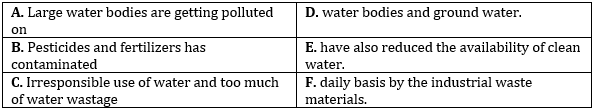(a) A-F, B-D and C-E
(b) A-F
(c) C-E
(d) C-E and B-D
(e) None of the above

Q9.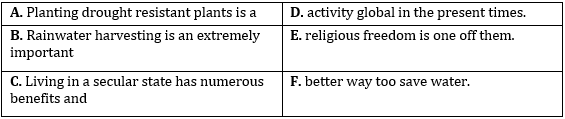(a) A-F, B-D and C-E
(b) A-F
(c) C-E
(d) C-E and B-D
(e) None of the above

Q10.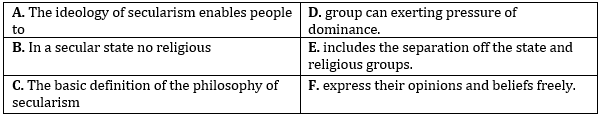(a) A-F, B-D and C-E
(b) A-F
(c) C-E
(d) C-E and B-D
(e) None of the above

Q11.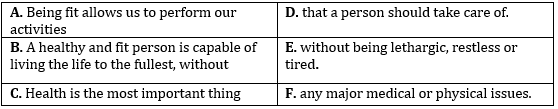(a) B-F
(b) A-E
(c) A-E and C-D
(d) A-E, B-F and C-D
(e) None of the above

Q12.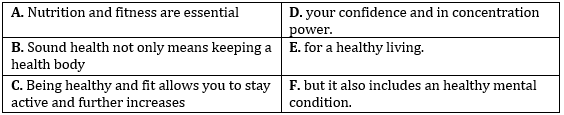(a) B-F
(b) A-E
(c) A-E and C-D
(d) A-E, B-F and C-D
(e) None of the above

Q13.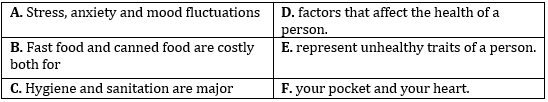(a) B-F
(b) A-E
(c) A-E and C-D
(d) A-E, B-F and C-D
(e) None of the above

Q14.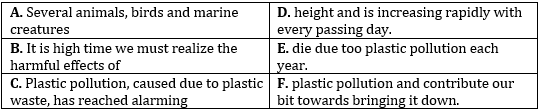(a) B-F
(b) A-E
(c) A-E and C-D
(d) A-E, B-F and C-D
(e) None of the above

Q15.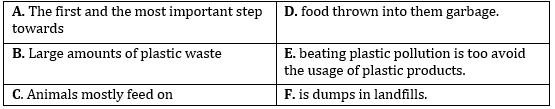(a) B-F
(b) A-E
(c) A-E and C-D
(d) A-E, B-F and C-D
(e) None of the above

Solutions

S1. Ans.(d)
Sol. ‘C-D’ is the correct combination here and makes a meaningful sentence.

S2. Ans.(c)
Sol. ‘A-E’ and ‘B-F’ are the correct combinations here and make meaningful sentences.

S3. Ans.(a)
Sol. ‘B-F’ is the correct combination here and makes a meaningful sentence.

S4. Ans.(b)
Sol. ‘A-E’ is the correct combination here and makes a meaningful sentence.

S5. Ans.(e)
Sol. None of the above

S6. Ans.(a)
Sol. ‘A-F’, ‘B-D’ and ‘C-E’ are the correct combinations here and make meaningful sentences.

S7. Ans.(d)
Sol. ‘B-D’ and ‘C-E’ are the correct combinations here and make meaningful sentences.

S8. Ans.(c)
Sol. ‘C-E’ is the correct combination here and makes a meaningful sentence.

S9. Ans.(e)
Sol. None of the above

S10. Ans.(b)
Sol. ‘A-F’ is the correct combination here and makes a meaningful sentence.

S11. Ans.(c)
Sol. ‘A-E’ and ‘C-D’ are the correct combinations here and make meaningful sentences.

S12. Ans.(b)
Sol. ‘A-E’ is the correct combination here and makes a meaningful sentence.

S13. Ans.(d)
Sol. ‘A-E’, ‘B-F’ and ‘C-D’ are the correct combinations here and make meaningful sentences.

S14. Ans.(a)
Sol. ” ‘B-F’ is the correct combination here and makes a meaningful sentence. In the case of ‘A-E’ it should be ‘to’ instead of ‘too’ and in the case of ‘C-D’ it should be ‘alarming heights’.”

S15. Ans.(e)
Sol. None of the above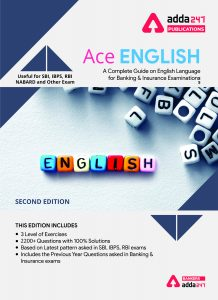Thank You, Your details have been submitted we will get back to you.